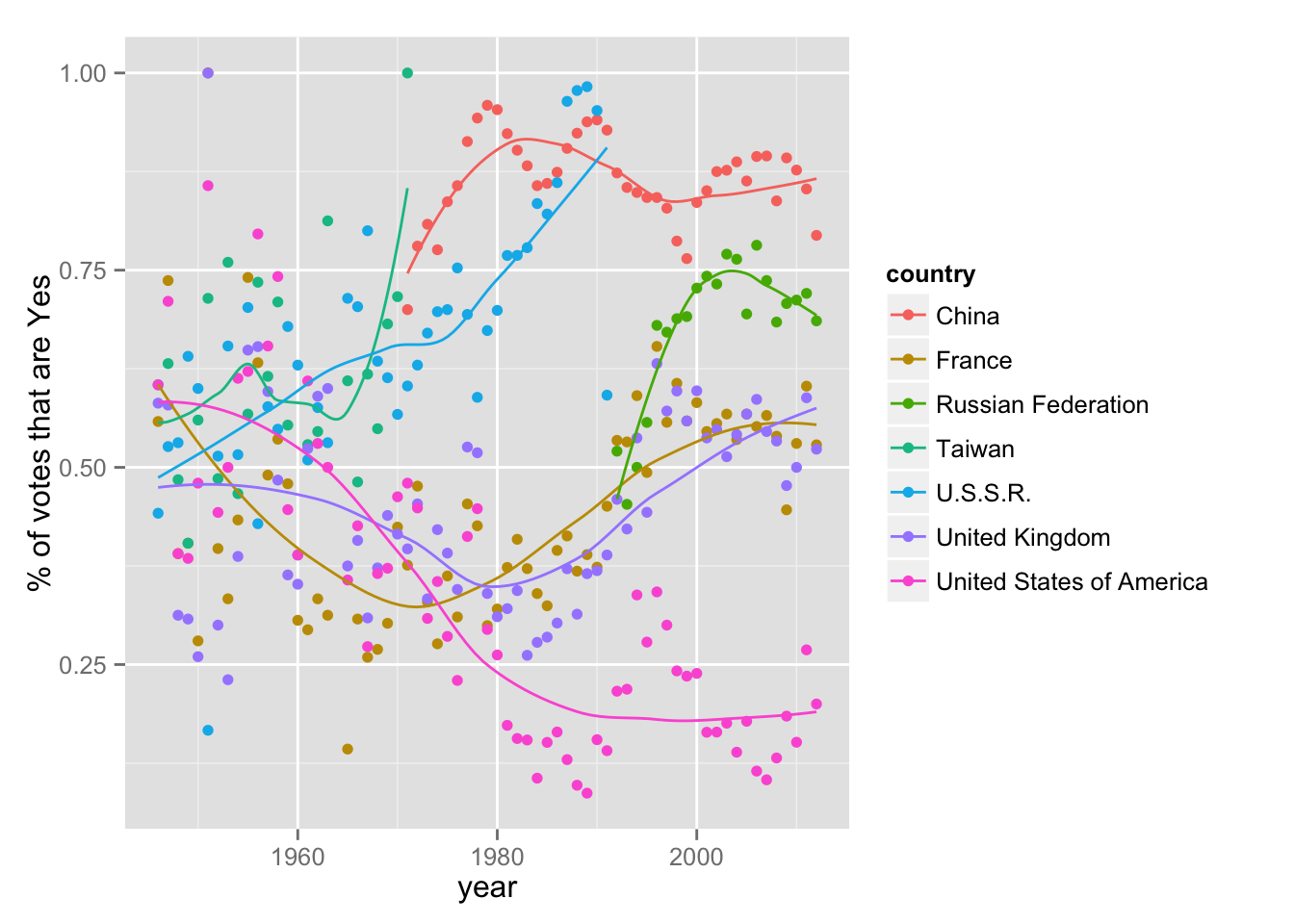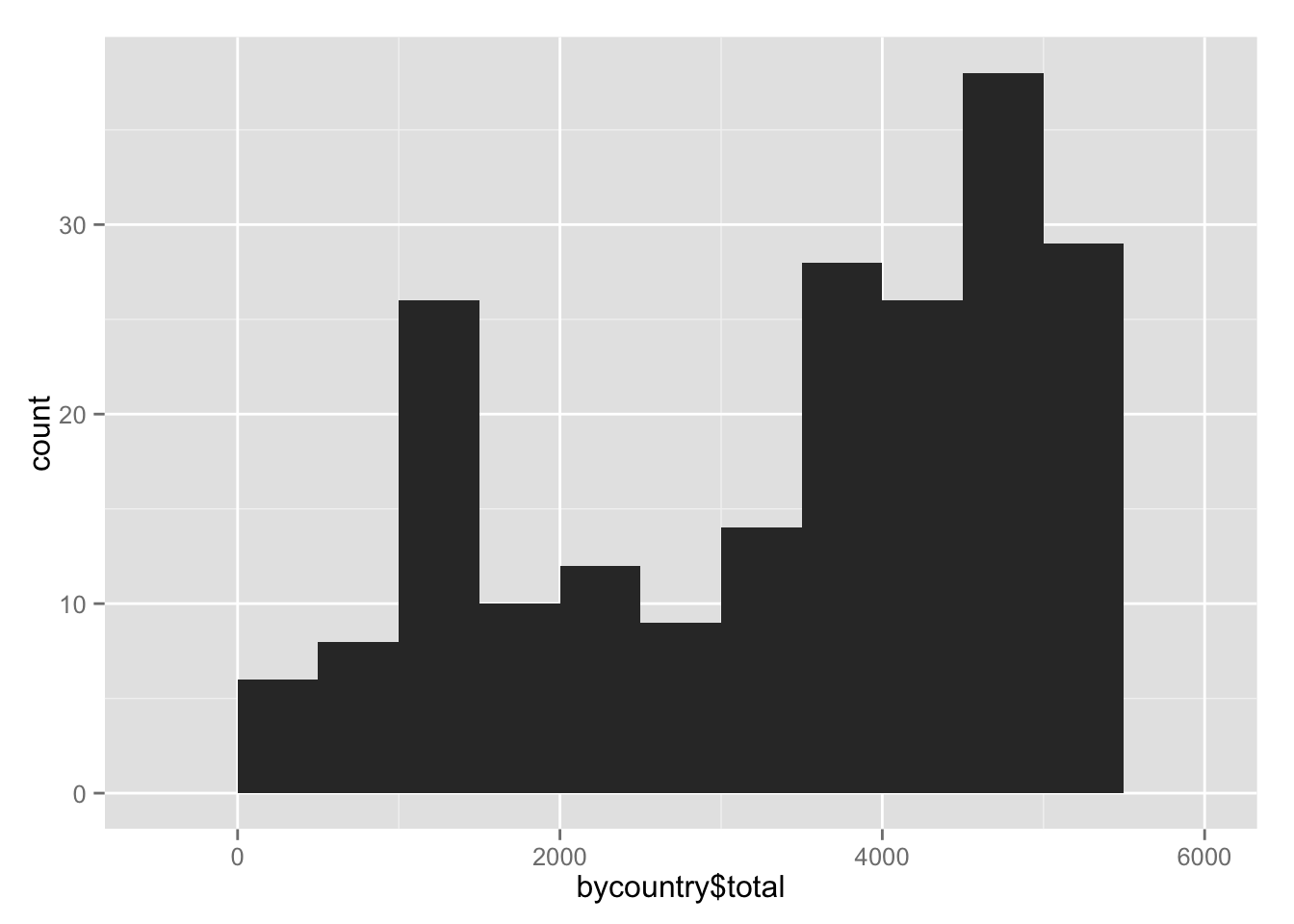Data was obtained in R format from Erik Voeten’s dataset here (specifically, under “Raw data”).

load("undata-213.RData")
YES <- 1
ABSTAIN <- 2
NO <- 3
ABSENT <- 8
NOTAMEMBER <- 9
library(plyr)
library(dplyr)
library(tidyr)

votes <- x %>% tbl_df() %>%
select(rcid, session, date, unres, vote, country = uniquename) %>% # just relevant columns
mutate(country = factor(gsub('"', '', country))) %>%
filter(vote %in% c(YES, NO, ABSTAIN)) %>%
separate(date, c("year", "month", "day"), sep = "-", remove = FALSE) %>%
mutate(year = as.numeric(year), month = as.numeric(month), date = as.numeric(date))

We look at the trends of the frequency of Yes votes over time.

byyear <- votes %>% group_by(country, year) %>%
summarize(yes = mean(vote == YES), no = mean(vote == NO))

# for now, consider the UN Security Council countries
countries <- c("United States of America", "United Kingdom", "U.S.S.R.",
"Russian Federation", "China", "France", "Taiwan")

library(ggplot2)
byyear %>% filter(country %in% countries) %>%
ggplot(aes(year, yes, color = country)) + geom_point() +
stat_smooth(se = FALSE) +
ylab("% of votes that are Yes")(Note that Taiwan became China, and the U.S.S.R. became the Russian Federation. Note also the wide gap that occurs at each of these transitions).

### Number of votes per country is bimodal

bycountry <- votes %>% group_by(country) %>% summarize(total = n())
qplot(bycountry$total, binwidth = 500)This suggests we should filter out countries with fewer Yes/No votes, which will add noise to our correlation measurement. manyvote_countries <- bycountry$country[bycountry\$total > 2000]

### voting blocs based on Manhattan distance of voting

library(reshape2)
votematrix <- votes %>% filter(country %in% manyvote_countries) %>%
acast(country ~ rcid, value.var = "vote")

# in new coding, 1 = YES, 0 = ABSTAIN, -1 = NO
votematrix <- 2 - votematrix
# construct a correlation matrix
cormat <- cor(t(votematrix), method = "spearman", use = "pairwise.complete.obs")
# replace missing values with 0 (not perfect!)
cormat[is.na(cormat)] <- 0
h <- hclust(dist(votematrix, method = "manhattan"))
plot(h, cex = .6)